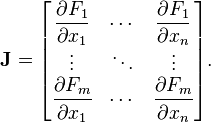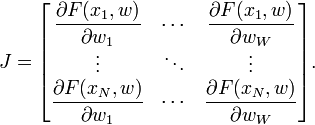2 views

Recently I started thinking about implementing the Levenberg-Marquardt algorithm for learning an Artificial Neural Network (ANN). The key to the implementation is to compute a Jacobian matrix. I spent a couple of hours studying the topic, but I can't figure out how to compute it exactly.

Say I have a simple feed-forward network with 3 inputs, 4 neurons in the hidden layer and 2 outputs. Layers are fully connected. I also have 5 rows of long learning sets.

1. What exactly should be the size of the Jacobian matrix?

2. What exactly should I put in place of the derivatives? (Examples of the formulas for the top-left, and bottom-right corners along with some explanation would be perfect)

This doesn't help:What are F and x in terms of a neural network?

by (108k points)

The Jacobian is a matrix of all the first-order partial derivatives of a vector-valued function. In the neural network case, it is an N-by-W matrix, where N is the number of entries in our training set and W is the total number of parameters (weights + biases) of our network. It can be generated by taking the partial derivatives of each output in respect to each weight, and has the form:Where F(xi, w) is the network function evaluated for the ith input vector of the training set using the weight vector w and wj is the jth element of the weight vector w of the network.

For more information regarding the Computation of the Jacobian matrix of a neural network in Python, refer to the following link: https://medium.com/unit8-machine-learning-publication/computing-the-jacobian-matrix-of-a-neural-network-in-python-4f162e5db180

In classical Levenberg-Marquardt implementations, the Jacobian is approximated by utilizing the finite differences. But, for neural networks, it can be computed very efficiently by using the chain rule of calculus and the first derivative of the activation functions.